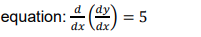# Write the sum of the order and the degree of the following differential equation:

CBSE Sample Question Paper, Class 12 Mathematics Term 2 Question - Write the sum of the order and the degree of the following differential equation:## Write the sum of the order and the degree of the following differential equation: